# Maharashtra Board 8th Science Topic -5 Inside The Atom solution

Q.1.Fill in the blank and rewrite the completed statements 18

1.Atoms of same element having different mass number are called …………… .

Ans Atoms of same element having different mass numbers are called isotopes.

2……..is used in the treatment of goiter, disease of thyroid gland.

Ans Iodine-131 is used in the treatment of goiter, disease of thyroid gland.

3………….is a machine that generates electricity on large scale using atomic energy.

Ans Nuclear reactor is a machine that generates electricity on large scale using atomic energy.

4.The maximum number of electrons a shell can contain is obtained by the formula …………… .

Ans The maximum number of electrons a shell can contain is obtained by the formula 2n2 .

4.Electron, proton, neutron are the types of in an atom.

Ans Electron, proton, neutron are the types of subatomic particles in an atom.

5……..is used for nuclear fission and production of electricity.

Ans Uranium-235 is used for nuclear fission and production of electricity.

1. The electron shell is nearest to the nucleus.

Ans The electron shell K is nearest to the nucleus.

1. are formed by chemical combinations of atoms.

Ans Molecules are formed by chemical combinations of atoms.

.8. …..is the smallest unit of matter.

Ans Atom is the smallest unit of matter.

9.The nucleus contains two types of subatomic particles together called …………… .

Ans The nucleus contains two types of subatomic particles together called nucleons.

10……….is the fundamental property of an element and its chemical identity.

Ans Atomic number is the fundamental property of an element and its chemical identity.

1. Atomic structure was studied at depth with the advent of a new branch of science called …………… .

Ans Atomic structure was studied at depth with the advent of a new branch of science called quantum mechanics.

12.The electron capacity of ‘L’ shell is …………… .

Ans The electron capacity of ‘L’ shell is eight.

1. An electron carries a charge.

Ans An electron carries a negative charge.

1. The valency of hydrogen is ‘one’ as per the molecular formula H2O. Therefore, valency of ‘Fe’ turns out to be…………… as per the formula Fe2O3.

Ans The valency of hydrogen is ‘one’ as per the molecular formula H2O. Therefore, valency of ‘Fe’ turns out to be three as per the formula Fe2O3.

1. The electrons in the outermost shell are called electrons.

Ans The electrons in the outermost shell are called valence electrons.

15.The electronic configuration of magnesium is 2,8,2. From this it is understood that the valence shell of Magnesium is …………… .

Ans The electronic configuration of magnesium is 2,8,2. From this it is understood that the valence shell of Magnesium is M.

1. Entire mass of the atom is concentrated in the …………… .

Ans Entire mass of the atom is concentrated in the nucleus.

# Q.2. Find the odd one out 8

1.Sodium-24, Carbon-13, Cobalt-60, Uranium-235

Ans : Carbon-13 – is the odd one out as it is not a radioactive element while the rests are radioactive.

2.Sodium, Hydrogen, Chlorine, Magnesium

Ans Magnesium – is the odd one out as it is bivalent while others are monovalent.

1. Ammonia, Sodium hydroxide, Methane, Chlorine

Ans Chlorine – is the odd one out as it an element while others are compound.

1. He, Xe, Br, Ar

Ans Br(Bromine) – is the odd one out as it not an inert gas while others are.

1. N, Na, Ne, Fe

Ans Ne(Neon) – is the odd one out as it is an inert gas element and will not form any compounds while others will form compounds.

Ans Graphite – is the odd one out as it is used as a moderator in nuclear reactor while others are used as controller.

1. Neutrons, Electrons, Nucleus, Protons

Ans Nucleus – is the odd one out as it is not the subatomic particle while others are.

8 Cl2, O2, CO2, H2.

Ans CO2 – is the odd one out as it is a compound while others are molecule of elements.

# Q.3. Find co-related terms 10

1 Chlorine : (2,8,1) :: Lithium : ……………

Ans Chlorine : (2,8,1) :: Lithium : (2, 1)

2 Nuclear fission : U-235 :: Cancer : ……………

Ans Nuclear fission : U-235 :: Cancer : Cobalt-60

3 Sodium : valency1 :: Nitrogen : ……………

Ans Sodium : valency1 :: Nitrogen : 3

4 ‘K’ shell : 2 electrons :: ‘M’ shell : ……………

Ans ‘K’ shell : 2 electrons :: ‘M’ shell : 18

5 Electron : Shell :: Neutron : ……………

Ans Electron : Shell :: Neutron : Nucleus 6 Carbon : (2,4) :: Oxygen : ……………

Ans Carbon : (2,4) :: Oxygen : (2,6)

1. Plum pudding model : J J Thomson :: Scattering experiment : ……………

Ans Plum pudding model : J J Thomson :: Scattering experiment : Rutherford

1. Methane : Compound :: Carbon : ……………

Ans Methane : Compound :: Carbon : Element 9 ‘L’ shell : 8 electrons :: ‘N’ shell : ……………

Ans ‘L’ shell : 8 electrons :: ‘N’ shell : 32

8. Age of archeological objects : C-14 :: Detection of cracks in underground pipes : ……………

Ans Age of archeological objects : C-14 :: Detection of cracks in underground pipes : Na-24

# Q.4. Extra data 4

1.Electron and Proton

## Ans

 Electron Proton Electron is a negatively charged subatomic particle. Proton is a positively charged subatomic particle

2.Thomson’s model and Rutherford’s model

Ans

 Thomson’s model Rutherford’s model According to Thomson’s model, an atom consists of a positively charged sphere with electrons embedded in it. According to Rutherford’s model, an atom consists of a positively charged particles concentrated at the Centre and the electrons revolve around the nucleus.

3.Proton and Neutron

Ans:

 Proton Neutron Proton is a positively charged subatomic particle. Neutron is an electrically neutral subatomic particle.

5.Rutherford’s model and Bohr’s model

## Ans

 Rutherford’s model Bohr’s model According to Rutherford’s model, an atom consists of a positively charged particles concentrated at the Centre and the electrons revolve around the nucleus. According to Bohr’s model, electrons revolving around the nucleus lie in the concentric circular orbits at certain distance from the nucleus.

# Q. 5. Match the pair 6

## 1.

 Column – A Column – B i. Uranium-235 a. Cancer ii. Iodine-131 b. Nuclear fission c. Goiter

Ans

 i. Uranium-235 Nuclear fission ii. Iodine-131 Goiter

## 2.

 Column – A Column – B i. Sodium-24 a. Cancer ii. Cobalt-60 b. Detection of cracks in pipes
 c. Age of archeological objects

Ans

 i. Sodium-24 Detection of cracks in pipes ii. Cobalt-60 Cancer

## 3

 Column – A Column – B i. Electron a. Neutral ii. Neutron b. Atoms c. Negatively charged

Ans

 i. Electron Negatively charged ii. Neutron Neutral

## 4

 Column – A Column – B i. Democritus a. Neutrons ii. Kanad Muni b. Atomos c. Paramanu

Ans

 i. Democritus Atomos ii. Kanad Muni Paramanu

## 5

 Column – A Column – B i. Proton a. Negatively charged ii. Electron b. Neutral c. Positively charged

Ans

 i. Proton Positively charged ii. Electron Negatively charged

## 6

 Column – A Column – B i. J J Thomson a. Scattering experiment ii. Rutherford b. Stable orbit atomic model c. Plum pudding model

Ans

 i. J J Thomson Plum pudding model ii. Rutherford Scattering experiment

# Q.7. State True or False 15

1.Energy of an electron is constant while it is in a particular orbit.

Ans True

1. The mass of an atom is mainly due to the protons and neutrons in its nucleus.

# Ans True

1. An atom as a whole is electrically neutral.

# Ans True

1. The mass of one hydrogen atom is approximately 1 u.

# Ans True

1. According to Rutherford, the positive charge is distributed throughout the atom and the negatively charged electrons are embedded in it.

Ans: False: According to J J Thomson, the positive charge is distributed throughout the atom and the negatively charged electrons are embedded in it.

1. The electrons in the outermost shell are called valence electrons.

Ans True

1. The indivisible particles that constitute matter were named by Kanad Muni as ‘Paramanu’.

Ans: True

8.The positive charge on the nucleus is due to the neutron in it.

Ans False:The positive charge on the nucleus is due to proton in it.

10.Negatively charged particles called electrons revolve around the nucleus.

Ans True

1. Electrons and protons are the two types of nucleons.

Ans False

1. Protons and Neutrons are the two types of nucleons. Atoms of all elements except inert gases have a non-zero valency.

## Ans True

1. Radioactive isotopes are used for food preservation from microbes.

Ans True

1. Isotopes have same atomic mass number but different atomic number.

Ans: False: Isotopes have same atomic number but different atomic mass number.

1. The ‘K’ shell has the maximum energy.

Ans False:The ‘K’ shell has minimum energy.

16.Cadmium is used as moderator for reducing the speed of neutrons in nuclear reactor.

Ans False

17.Graphite is used as moderator for reducing the speed of neutrons in nuclear reactor.

# Q.7. Name the following 19

1.An electrically negative subatomic particle.

Ans Electrons

2.An electrically neutral subatomic particle.

Ans Neutrons

3.The plum pudding model of atom was put forth by.

Ans J J Thomson

4.An element having valency ‘zero’.

Ans Element He, Ne, Ar, Xe

1. Element used as a moderator for reducing the speed of neutrons in nuclear reactor.

Ans Graphite

5.The shell closest to the nucleus of an atom.

Ans ‘K’ shell

1. The symbol and the ordinal number of the inner most shell.

Ans Symbol for the inner most shell is ‘K’. Ordinal number(n) for the inner most shell is one.

1. First nuclear reactor in India.

Ans ‘Apsara’at Bhabha Atomic Research Centre in Mumbai

1. Isotope used for the treatment of Cancer.

Ans Cobalt-60

1. Isotope used for the production of energy.

Ans Uranium-235

10.The three isotopes of hydrogen.

Ans Protium 1 1H, Deuterium 2 1H , Tritium 3 1H

11.Element used as controller in nuclear reactor.

12.An electrically positive subatomic particle.

Ans Protons

1. A symbol used for the ordinal number of a shell.

Ans ‘n’

1. Electronic configuration of helium atom.

Ans (2)

1. The valency of an element having electronic configuration (2, 8, 5)

Ans three

16.Valency of chlorine atom

Ans one

1. The smallest unit of matter.

Ans atom

1. Elements having same atomic number but different atomic mass number.

Ans Isotopes

# Q.7.Answer in one sentence 23

1.Define : Isotope

Ans Atoms of same element having same atomic number but different atomic mass number are called isotopes.

1. What are the symbols used for the shells which accommodate the electrons in various atoms?

Ans The symbols used for the shells are K, L, M, N.

1. Which is the outermost shell of hydrogen atom?

Ans The outermost shell of hydrogen atom is ‘K’ as it electronic configuration is (1) and it has only one shell.

4.There is a similarity in the atomic structure and solar system. The planets revolve around the sun due to the gravitational force. Which force might be acting in the atomic structure?

Ans In the atomic structure, the nuclear force keeps the electron revolving in fixed orbits around the nucleus.

5.Which is the outermost shell of sodium atom?

Ans The outermost shell of sodium atom is ‘M’ as its electronic configuration is (2,8,1)

1. Where are electrons revolving around the nucleus placed?

Ans Electrons are revolving around the nucleus in specific orbits called ‘shells’.

1. What is the symbol and ordinal number of the inner most shell?

Ans i. The symbol of the inner most shell is K.

1. Ordinal number of the innermost shell is 1.

2. Define : Moderator in nuclear reactor

Ans To control the chain reaction in the nuclear reactor, it is necessary to decrease the speed and number of neutrons. Graphite or heavy water is used as moderator for reducing the speed of neutrons.

10.Write symbol of electronic distribution in shell of fluorine atom?

Ans i. The electronic configuration of fluorine atom having atomic number 9 is (2,7)Therefore, its electronic distribution is K,L

11.The atomic number and mass number of magnesium are 12 and 24 respectively. How will you show this by the convention symbol?

Ans i. Atomic number of Magnesium (A) = 12

1. Atomic mass number (Z) = 24 iii.Conventional symbol for Magnesium= ASymbol

Z= 24 Mg 12

1. Define : Nuclear Reactors

Ans Nuclear reactor is a machine that generates electricity on large scale by using atomic energy.

15.Write the electronic configuration of the following. 27 X

Ans :27 X 13

Atomic number = 13 (number of protons=number of electrons=13)

∴ electronic configuration= 2,8,3

1. Which is the outermost shell of fluorine atom?

Ans The outermost shell of fluorine atom is ‘L’ as its electronic configuration is (2,7).

1. Define : Atomic mass number (A)

Ans Atomic mass number is the total number of protons and neutrons present in an atom.

18.What is meant by matter?

Ans Matter is anything around us that we can perceive through our senses. It is made up of particles.

19.Which subatomic particles are present in the nucleus?

Ans Protons and neutrons are present in the nucleus of an atom.

1. Define: Atom

Ans An atom is the smallest particle of an element which retains its chemical identity in all the physical and chemical changes.

1. Dalton’s and Thomson’s atomic models?

Ans In Rutherford’s atomic model, positively charged nucleus is present in the centre of an atom. While nucleus is not present in the Dalton’s and Thomson’s model.

1. Define : Electronic configuration of the element

Ans The shell wise distribution of the electron in an atom of an element is called the electronic configuration of the element.

1. How many types of subatomic particles are found in atom?

Ans There are three types of subatomic particles namely protons, electrons and neutrons.

1. Define : Atomic number (Z)

Ans Atomic number is the number of protons or electrons present in an atom.

1. Which subatomic particles are electrically charged?

Ans Protons and electrons are electrically charges. Protons are positively charged while electrons are negatively charged.

# Q.8. Solve Numerical problems: 2

1 The atomic number and mass number of calcium are 20 and 40 respectively. Deduce the number of neutrons present in the calcium nucleus.

Ans i. Atomic number (Z) of calcium = 20

1. Atomic mass number (A) of calcium = 40
2. Number of protons = Number of electrons = 20 (Z= number of protons)
3. Number of neutrons = Atomic mass number – Number of protons

= 40 – 20

= 20

1. ∴ Number of neutrons = 20

# Q.8. Write Short Notes 4

1. Isotopes

Ans i. Some elements in nature have atoms with same atomic number but different mass number.

1. Such atoms of the same element having different mass number are called isotopes.
2. For example, carbon has three isotopes namely, C-12, C-13, C-14. The mass number of isotopes is also represented by another method as 12C, 13C and .14C. The isotopes have same proton number but different neutron number.
3. Nuclear Reactor

Ans i. Nuclear reactor is a machine that generates electricity on large scale by using atomic energy

1. In a nuclear reactor, the nuclear energy in atom is released by bringing about nuclear reactions on the nuclear fuel
2. In a nuclear reaction, when Uranium-235 is bombarded with slow speed of neutrons, the nucleus of the isotope Uranium-235 undergoes nuclear fission to form nuclei of two different elements Krypton-92 and Barium-141 and 2 to 3 neutrons.
3. On decreasing the speed these neutrons bring about fission of more Uranium-235 nuclei.
4. In this way a chain reaction of nuclear fission takes place.
5. A large amount of energy is released during a chain reaction of fission.
6. The chain reaction is kept under control to prevent the probable explosion. viii.The heat produced in the fission process is taken out by using water as coolant.

ix. Water is transformed into steam. By means of this heat, turbines are driven and electricity is generated.

# Q.9. Complete the given flow chart / table / diagram 2

1 Write down the number of electrons present in each of them.

 Element H C Na Cl N Z 1 6 11 17 7 Number of electrons in the outermost shell ….. ….. ….. ….. …..

# Ans

 Element H C Na Cl N Z 1 6 11 17 7 Number of electrons in the outermost shell 1 4 1 7 5

Q.10. Distinguish between 4

1. Electron and Proton

# Ans

 Electron Proton Electron is a negatively charged subatomic particle. Proton is a positively charged subatomic particle. Electrons revolve in orbits around the nucleus. Protons are present in the nucleus of an atom. The mass of an electron is negligible. The mass of a proton is approximately equal to 1u(1 Dalton) An electron is represented by the symbol ‘e’. A proton is represented by the symbol ‘p’.

Q11. Proton and Neutron

## Ans

 Proton Neutron Proton is a positively charged subatomic particle. Neutron is an electrically neutral subatomic particle. In an atom, the number of protons is equal to the number of electrons. In an atom, the number of neutrons may be or may be equal to the number of electrons. A proton is represented by the symbol ‘p’. A neutron is represented by the symbol ‘n’.

Q.12. Give scientific reasons 10

1. Atom is electrically neutral.

Ans i. In an atom of an element, the number of electrons in the outer orbits is equal to the number of protons in the nucleus.

Protons are positively charged while the electrons are negatively charged and neutrons are electrically neutral.

The total number of protons is equal to the total number of electrons.

Therefore, electrical charges are balanced and the atom as a whole is electrically neutral.

2. All the mass of an atom is concentrated in the nucleus.

Ans i. In an atom of an element, protons and neutrons are present in the atomic nucleus while the electrons revolve around the nucleus in different orbits.

1. The mass of a proton is approximately equal to 1 Dalton and is also equal to the mass of a neutron.
2. The mass of an electron is 1800 times less than that of a hydrogen atom and hence is negligible compared to that of the proton and neutron.
3. Therefore, in an atom, all the mass is concentrated in the nucleus
4. Atoms are stable though negatively charged electrons are revolving within it.

Ans i. According to the Bohr’s atomic model, the electrons revolving around the atomic nucleus lie in the

3. concentric circular orbits at certain distance from the nucleus.

Energy of an electron is constant while it is in a particular orbit.

When an electron jumps from one orbit to another, it either absorbs or emits energy equal to the difference of its energy level.

Hence, atoms are stable though negatively charged electrons are revolving within it.

4. If sodium metal is put in water, it burns to give yellow flame.

Ans i. If sodium metal is put in water, it burns to give yellow flame because the electron of sodium absorbs energy and goes to outermost shell and come back to inner shell by emitting energy.

ii. The difference .of energy level of these two shells of sodium is fixed. iii.The difference is similar to energy of yellow light.

iv.For example: On road sodium vapor lamp gives yellow color light.

5. Atomic mass number is a whole number.

Ans i. Atomic mass number is the total number of protons and neutrons present in an atom.

The mass number is denoted by the symbol ‘A’.

Number of protons and neutrons in the nucleus are always a whole number and not a fraction.

Hence, atomic mass number is a whole number.

# Q.13..Draw / Label the diagram 8

1. Diagrammatic sketch of electronic configuration of Magnesium

#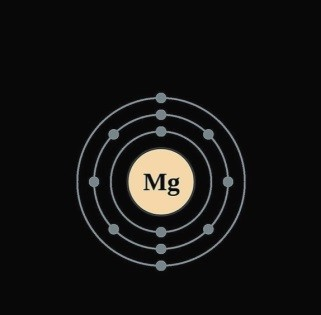## Ans:

1.Thomson’s atomic model.

#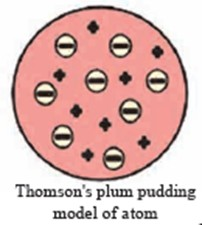## Ans

2.Rutherford’s scattering experiment.

#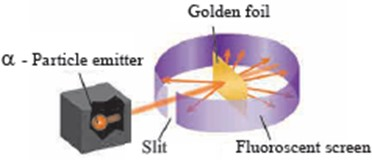Ans

3.Diagrammatic sketch of electronic configuration of H, He, C, Ne, Na

## Ans: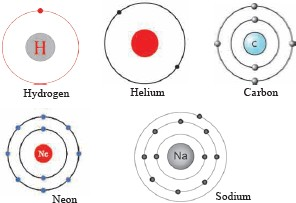Q.14.Complete the table/ web/ flow chart/diagram/paragraph 9

1 The number of electrons of some elements is given here. By using it write the electronic configuration, number of valence electron and valency of the respective elements.

 Element Li C Mg O Number of electrons 3 6 12 8 Electronic configuration ….. ….. ….. ….. Number of valence electrons ….. ….. ….. ….. Valency ….. ….. ….. …..

## Ans

 Element Li C Mg O Number of electrons 3 6 12 8 Electronic configuration 2, 1 2, 4 2, 8, 2 2, 6 Number of valence electrons 1 4 2 6 Valency 1 4 2 b

2

 Isotopes Proton number Neutron number 1 H 1 …………… …………… 2 H 1 1 1 3 H 1 1 2 35Cl 17 …………… …………… 35Cl 17 …………… ……………

## Ans

 Isotopes Proton number Neutron number Protium 1H 1 1 0 Deuterium 2H 1 1 1 Tritium 3H 1 1 2 35Cl 17 17 18 35Cl 17 17 20

3 Deduce from the datum provided

 Datum To deduce Answer 23 Na 11 Neutron number …………… 12 C 6 Mass number ……………
 37 Cl 17 Proton number ……………

## Ans

 Datum To deduce Answer 23 Na 11 Neutron number 23 – 11 = 12 12 C 6 Mass number 12 37 Cl 17 Proton number 17

Q.15. Write answers based on given diagram/paragraph 3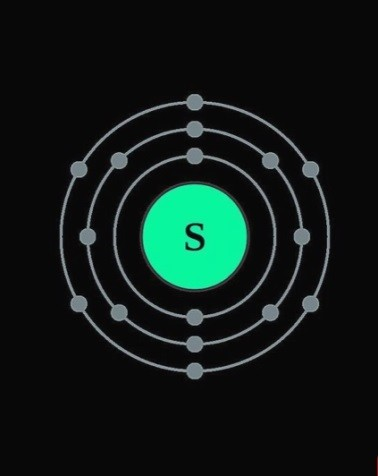1 Observe the diagram and answer the following.

a.Write the electronic configuration of the element? b.State the valency of the element.

c.State the atomic number of the element. d.Write the electron distribution for the element?

Ans a.The electronic configuration of the element is (2,8,6) b.The valency of the element is 2.

c.The atomic number of the element is 16 d.The electron distribution is K, L, M

1.Write the number of neutrons in the nucleus of an atom having atomic number 15 and mass number 31.

Ans i. Atomic number = 15 (number of protons = 15)

Mass number = 31

Number of neutrons = Mass number – number of protons

= 31 – 15 = 16

∴ Number of neutrons = 16

2. What is heavy water?

Ans i. Heavy water, (formally called deuterium oxide) is the water in which hydrogen atoms have been replaced by deuterium.

ii. The hydrogen-1 isotope (Protium) makes up most of the hydrogen in normal water. iii.Heavy water is used as a moderator in certain nuclear reactors.

3.What is the difference in the atomic models of Thomson and Rutherford?

Ans i. According to the Thomson’s model of an atom, the positive charge is distributed throughout the atom and the negatively charged electrons are embedded in it.

According to Rutherford’s nuclear model for atom, the positively charged nucleus is at the centre of the atom and negatively charged particles called electrons revolve around the nucleus.

Thus, Thomson’s model does not state any details about the nucleus while Rutherford’s model of atom states the presence of nucleus in the centre of the atom.

4. What is meant by atomic mass number? Explain how the atomic number and mass number of carbon are 6 and 12 respectively.

Ans i. The total number of protons and neutrons in an atom is the atomic mass number of that element. The mass number is denoted by the symbol ‘A’.

Atomic number is the number of electrons or protons present in an atom. Atomic number is denoted by the symbol ‘Z’.

For a Carbon atom, the mass number and atomic number is denoted as 126C.

There are 6 protons and 6 electrons in the carbon atom and hence its atomic number is 6.

Atomic mass number of Carbon atom is 12 and so there are 6 neutrons (12-6) in its nucleus.

5. State Dalton’s Atomic theory.

Ans i. John Dalton put forth his celebrated ‘Atomic Theory’ in 1803 A.D.

ii. According to this theory, matter is made of atoms and atoms are indivisible and indestructible. iii.All atoms of an element are alike while different elements have different atoms with different mass.

iv.The mass is distributed uniformly in an atom.

6.State the important postulates of Bohr’ atomic model.

Ans The important postulates of Bohr’s atomic model are as follows:

The electrons revolving around the atomic nucleus lie in the concentric circular orbits at certain distance from the nucleus.

Energy of an electron is constant while it is a particular orbit.When an electron jumps from an inner orbit to an outer orbit it absorbs energy equal to the difference of its energy level and when it jumps from an outer orbit to an inner orbit it emits energy equal to the difference of it energy level.

7.Which discovery did point out that an atom has internal structure?

Ans i. Thomson’s theory pointed out that an atom has internal structure consisting of positive charges that are evenly distributed and negative charges are embedded in it.

ii.And Rutherford’s scattering experiment proved that atom consists of nucleus and electrons revolve around them.

8.Describe the outcomes of the Rutherford’s scattering experiment.

Ans i. Rutherford observed that the large number of the α-particles that went straight through the foil indicates that there was no obstacle in their path. It means that there must be empty space inside the atoms in the solid gold foil.

The small number of α-particles that got deflected through a small or a big angle must have faced an obstacle in their path. It meant that the positively charges and heavy part causing obstruction would be in the centre of the atom.

Based on these observations, Rutherford put forth a nuclear model for atom.

9. Explain the difference between Thomson’s and Rutherford’s atomic model on the basis of

Distribution of positive charges.

Position of electrons.

###### Ans a. Distribution of positive charges –

According to the Thomson’s atomic model, the positive charges are distributed throughout the atom whereas according to the Rutherford’s model, there is a positively charged nucleus at the centre of the atom.

## b. Position of electrons –

According to the Thomson’s atomic model, the negatively charged electrons are embedded in the positive charges and the distributed positive charge is balanced by the negative charge on the electrons. Whereas in Rutherford’s atomic model, the negatively charged particles called electrons revolve around the nucleus which is positively charged.

10.Sulphur contains 16 protons and 16 neutrons. What would be its atomic number and mass number?

Ans i. Given :

Number of protons = 16

Number of electrons= 16

Number of neutrons = 16

Atomic number(Z) of Sulphur is 16 (Atomic number = no of protons)

Atomic mass number (A) of Sulphur = 16 + 16 = 32 (A= No of protons + No of neutrons)

11.Explain Rutherford’s nuclear atomic model.

Ans According to Rutherford’s nuclear atomic model:

There is a positively charged nucleus at the centre of an atom.

Almost the entire mass of the atom is concentrated in the nucleus.

Negatively charged particles called electrons revolve around the nucleus.

The total negative charge on all the electrons is equal to the positive charge on the nucleus. As the opposite charges are balanced the atom is electrically neutral.

There is an empty space between the revolving electron and the atomic nucleus.

12. State the provisions made in the nuclear reactor to prevent explosion.

Ans i. Nuclear reactor is a machine that generates electricity on large scale by using atomic energy.

The chain reaction is kept under control by decreasing the speed and number of neutrons to prevent explosion.

For this purpose, the following provision is made in a nuclear reactor.

Moderator:Graphite or heavy water is used as moderator for reducing the speed of neutrons.

Controller:Rods of boron, cadmium, beryllium, etc. are used as controller to reduce the number of neutron by absorbing them.

13.Atomic number of carbon is 6. How many electrons are there in a carbon atom?

Ans i. Atomic number of carbon (Z) = 6 (Z = number of protons)

Number of protons = 6

∴ number of electrons = 6 (number of protons= number of electrons)

14. A sodium atom contains 11 electrons. What is the atomic number of sodium?

Ans i. Number of electrons = 11

ii.Atomic number of sodium (Z) = 11 (Z = number of protons & number of protons = number of electrons)

Explain Thomson’s plum pudding model with the help of a neat labelled diagram.

Ans i. The plum pudding model of atom put forth by Thomson in the year 1904 is the first model of atomic structure.

According to this model, the positive charge is distributed throughout the atom and the negatively charged electrons are embedded in it.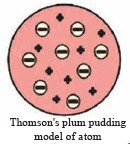The distributed positive charge is balanced by the negative charge on the electrons. Therefore, the atom becomes electrically neutral.

15.What is the difference between the solid atom in Dalton’s atomic theory and Thomson’s atomic model?

Ans i. According to Dalton’s theory, an atom is a hard, solid sphere with no internal structure. And that atom is indivisible and indestructible.

ii.While according to Thomson’s plum pudding model, the positive charge is distributed throughout the atom and the negatively charges electron are embedded in it.

16.Positively charged protons are together in the nucleus. What might be, one of the function of the neutrons in the nucleus?

Ans i. Neutrons play a very important role in an atom.

ii. They provide stability to the atom and also prevent protons from repelling one another. iii.Neutrons also add mass to the atom.

17.The symbol used for oxygen is ‘O’. There are 8 protons and 8 neutrons in its nucleus. From this determine the atomic number (Z) and mass number (A) of oxygen and arrange these in a conventional symbol.

Ans i. Given

Number of protons = 8

Number of electrons = 8 (p=e)

Number of neutrons = 8

Atomic number (Z) of oxygen = 8 (Z=Number of proton)

Atomic mass number (A) of oxygen = Number of protons + Number of neutrons

= 8 + 8 = 16

A = 16

Conventional symbol for oxygen = A Symbol

Z

= 16O

8

# Q. 17.Read the given paragraph and answer questions based on it 5

1 Valency means the number of chemical bonds formed by an atom. Valency of an element remains constant in its compound. Atom forms chemical bonds by using electron of its outermost shell. Valency of an atom is determined by the configuration of its outermost shell. Therefore the outermost shell is called valence shell. Also, the electrons in the outermost shell are called valence electrons. Valency is related to the number of valence electrons in that atom.

i.What is valency?

ii.How is the valency of an atom determined?

iii.If an element has 6 valence electrons, then what is the valency of that element?

iv. If an element ‘X’ forms a bond with chlorine atom and the compound formed is v.. What is the valency of that element ‘X’.

Ans i. Valency means the number of chemical bonds formed by an atom.

ii.Valency of an atom is determined by the configuration of its outermost shell.

iii.If an element has 6 valence electrons, then the valency of that element is 2, as it requires 2 more electrons to complete its octet.

iv. The valency of the element is One.

# Q.18. Complete the given diagram/ table / flowchart/ paragraph 20

1

 Sr. No. Symbol of element Molecular formula of compound Valency of the element Electronic configuration of the element Number of valence electrons x 8-x (for x= or > 4) 1 H HCl 1 1 1 … 2 Cl HCl 1 2, 8, 7 7 8 – 7 = 1 3 Ne No compound 0 4 F HF 5 Na NaH 6 Mg MgCl2 7 C CH4 8 Al AlCl3

## Ans

 Sr. No. Symbol of element Molecular formula of compound Valency of the element Electronic configuration of the element Number of valence electrons x 8-x (for x= or > 4) 1 H HCl 1 1 1 … 2 Cl HCl 1 2, 8, 7 7 8 – 7 = 1 3 Ne No compound 0 2,8 0 8 – 8 = 0 4 F HF 1 2, 7 7 8 – 7 = 1 5 Na NaH 1 2, 8, 1 1 … 6 Mg MgCl2 2 2, 8, 2 2 … 7 C CH4 4 2, 4 4 8 – 4 = 4 8 Al AlCl3 3 2, 8, 3 3 …

2

 Shell Electron capacity of the shell Symbol n Formula: 2n2 Number of electrons K 1 2 x (1)2 …………… L ….. …………… …………… M ….. …………… …………… N ….. …………… ……………

## Ans

 Shell Electron capacity of the shell Symbol n Formula: 2n2 Number of electrons K 1 2 x (1)2 2 L 2 2 x (2)2 8 M 3 2 x (3)2 18 N 4 2 x (4)2 32

3

 Atom Symbol Electron number in the atom Distribution of electrons Electronic configuration in the numerical form Shell symbol(maximum capacity) K (2) L(8) M(18) N(32) Lithium Li 3 2 1 2, 1 Oxygen O 8 Fluorine F 9 Neon Ne 10 Chlorine Cl 17 Argon Ar 18

## Ans

 Atom Symbol Electron number in the atom Distribution of electrons Electronic configuration in the numerical form Shell symbol(maximum capacity) K (2) L(8) M(18) N(32) Lithium Li 3 2 1 2, 1 Oxygen O 8 2 6 2, 6 Fluorine F 9 2 7 2, 7 Neon Ne 10 2 8 2, 8 Chlorine Cl 17 2 8 7 2, 8, 7 Argon Ar 18 2 8 8 7 2, 8, 18, 7

4

 Atom Symbol Electron number in the atom Distribution of electrons Electronic configuration in the numerical form Shell symbol(maximum capacity) K (2) L(8) M(18) N(32) Hydrogen H 1 1 1 Helium He 2 2 2 Carbon C 6 Nitrogen N 7 Bromine Br 35 Sodium Na 11

## Ans

 Atom Symbol Electron number in the atom Distribution of electrons Electronic configuration in the numerical form Shell symbol(maximum capacity) K (2) L(8) M(18) N(32) Hydrogen H 1 1 1 Helium He 2 2 2 Carbon C 6 2 4 2, 4 Nitrogen N 7 2 5 2, 5 Bromine Br 35 2 8 18 7 2, 8, 18, 7 Sodium Na 11 2 8 1 2, 8, 1

Q.19. Answer the following in detail 30

1.What is meant by valency of an element? What is the relationship between the number of valence electrons and valency?

Ans i. Valency means the number of chemical bonds formed by an atom.

Valency of an atom is determined by the configuration of its outermost shell.

Therefore, the outermost shell is called the valence shell and the electrons in the outermost shell are called valence electrons.

Thus, valency of an element is same as the number of its valence electrons, if this number is four or less than four.

On the other hand, when an element has four or more valence electrons, the number of electron by which the octet is short of completion is the valency of that element.

For example: For Sodium atom having .electronic configuration (2,8,1), the valency is one because it will give away one electron to achieve a stable octet state

Similarly, For oxygen having electronic configuration (2,6), the valency is two because it needs two more electrons to achieve a stable octet.

## Four elements A,B, C and D are given

A shows the presence of 20 neutrons, 17 protons and 17 electrons. B shows the presence of 10 neutrons, 10 protons and 10 electrons. C shows the presence of 18 neutrons, 17 protons and 17 electrons. D shows the presence of 8 neutrons, 7 protons and 7 electrons.

# State which of the above is

1.An element with zero valency

2.A pair of isotopes

3.An element with mass number 15

Ans i. An element with zero valency is ‘B’ a.Number of electrons for element ‘B’ is 10 b.Electronic configuration is 2,8

c.So it has stable electronic octet and hence its valency is zero.

# A pair of isotopes is elements ‘A’ and ‘C’.

a.Isotopes are elements having same atomic number but different atomic mass number. b.Elements A and C have same atomic number (Z = 17).

c.But different neutron number and hence their mass number is different. d.Mass number of element A = 20 + 17 = 37.

e.Mass number of element C = 18 + 17 = 35.

f. 37 A and 35 C are pair of isotopes.

17 17

## An element with mass number 15 is the element ‘D’

a.Number of protons for element ‘D’ is 7 b.Number of neutrons for element ‘D’ is 8

c.Mass number (A) = 7+8= 15

1. Complete the table by putting tick marks in appropriate box to indicate the information of the smallest particle and the type of matter.
 Name of substance Formula Smallest particle of the substance Type of matter Is an atom Is a molecule Single type of atoms in the molecule Different types of atoms in the molecule Element Compound Helium He √ √ √ Methane CH4 Argon Ar Neon Ne Chlorine Cl2

## Ans

 Name of substance Formula Smallest particle of the substance Type of matter Is an atom Is a molecule Single type of atoms in the molecule Different types of atoms in the molecule Element Compound Helium He √ √ √ Methane CH4 √ √ √ Argon Ar √ √ Neon Ne √ √
 Chlorine Cl2 √ √ √
1. What is meant by subatomic particle? Give brief information of three subatomic particles with reference to electrical charge, mass and location.

Ans i. Subatomic particle is a unit matter that is smaller than an atom or found inside an atom

ii. The three subatomic particles are proton, electron and neutron respectively.

## Proton:

Proton is a positively charged subatomic particle in the atomic nucleus and is represented by the symbol ‘p’.

Each proton carries a positive charge of +1e (1e= 1.6 x 10-19 coulomb)

Mass of one proton is approximately 1 u (Dalton).

# Neutron:

Neutron is an electrically neutral subatomic particle in the atomic nucleus and is represented by the symbol ‘n’.

Neutrons do not carry any charge.

The mass of a neutron is approximately 1 u (Dalton), which is almost equal to that of a proton.

# Electron:

Electron is a negatively charged subatomic particle and is represented by the symbol ‘e’.

Electrons revolve in the discrete orbits around the nucleus.

Each electron carries one unit of negative charge (-1e).

Mass of an electron is 1800 times less than that of a hydrogen atom. Therefore, the mass of an electron can be treated as negligible.

.Explain Rutherford’s scattering experiment with a labelled diagram.

Ans i. Rutherford took a very thin gold foil(10-4 mm) and bombarded it with positively charged α-particles emitted by a radioactive element.

He observed the path of α-particles by means of a fluorescent screen around the gold foil.

It was expected that the α-particles would get reflected from the gold foil if the positively charged mass were evenly distributed inside the atoms.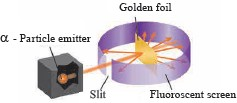Unexpectedly, most of the α-particles went straight through the foil, a small number of α-particles got deflected through a small angle, a still small number of α-particles get deflected throughout a larger angle and surprisingly one α-particle out of 20000 bounced back in the direction opposite to the original path.

State the uses of Isotopes.

Ans The uses of isotopes are as follows:

Uranium-235 is used for nuclear fission and production of electricity.

Some radioactive isotopes like Cobalt-60 are used in the medical treatment of fatal diseases like cancer. iii.Iodine-131 is used in the treatment of goiter, disease of thyroid gland.

The radioactive isotopes are used for detection of cracks (leakage) in the underground pipes. Eg. Sodium- 24.

Radioactive isotopes are used for food preservation from microbes.

The radioactive isotope C-14 is used for determining the age of archeological objects.

This site uses Akismet to reduce spam. Learn how your comment data is processed.